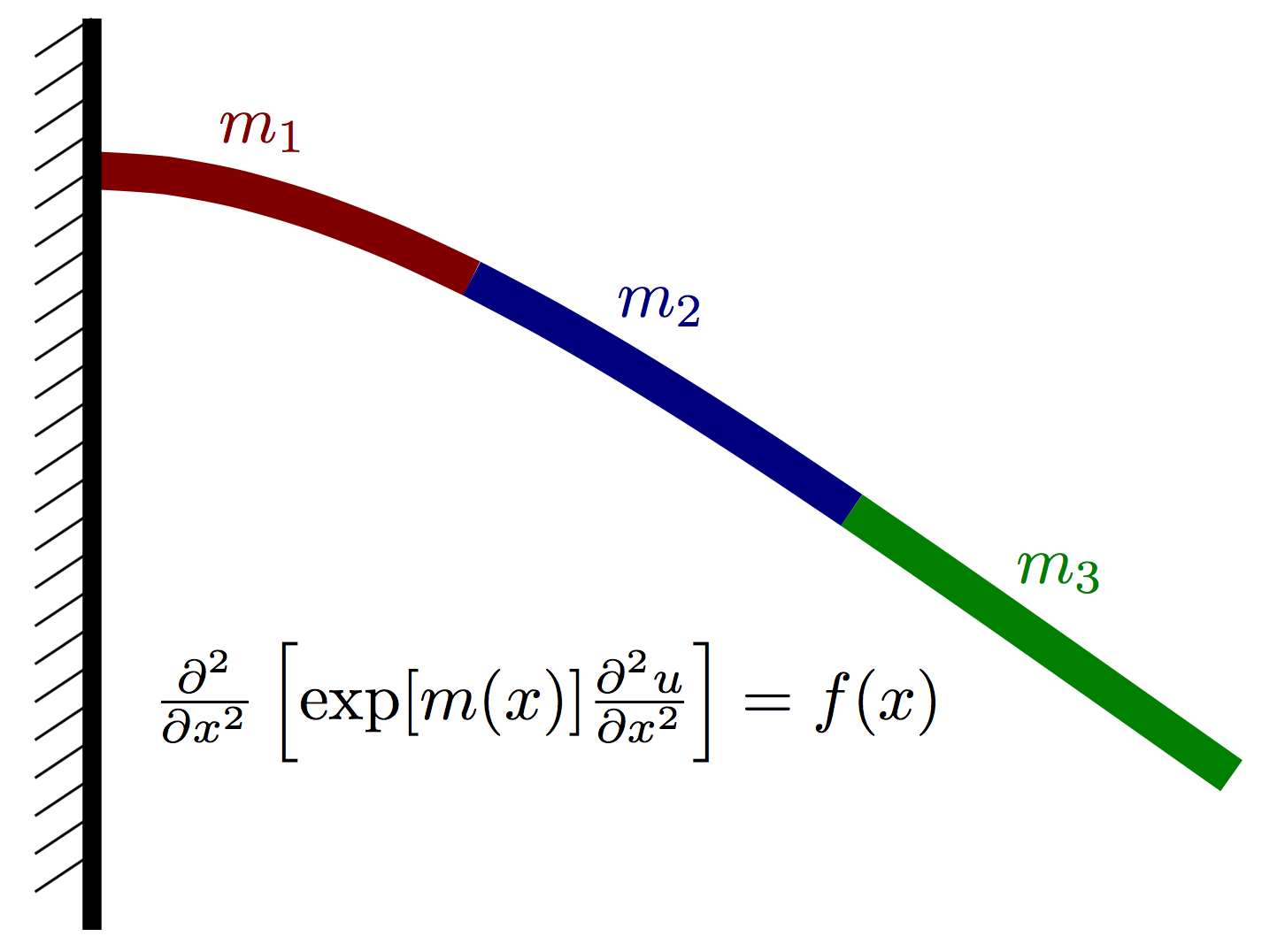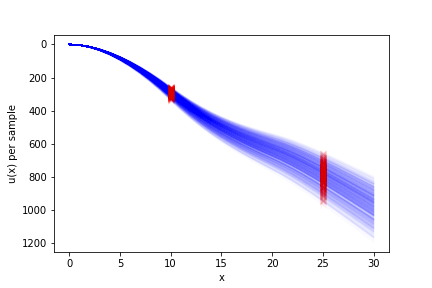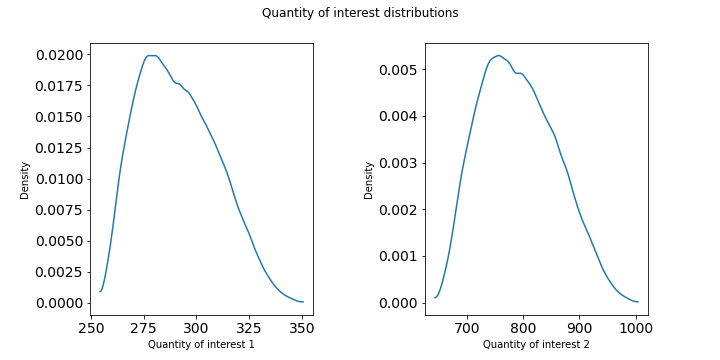# Uncertainty propagation of material properties of a cantilevered beam¶

## Overview¶

This is an uncertainty propagation problem modeling the effect of uncertain material parameters on the displacement of an Euler-Bernoulli beam with a prescribed load.

The Young’s modulus is piecewise constant over three regions as shown below, and the three constants are assumed to each be uncertain with a uniform distribution.## Run¶

docker run -it -p 4243:4243 linusseelinger/benchmark-muq-beam-propagation:latest


## Properties¶

Model

Description

forward

Forward model

### forward¶

Mapping

Dimensions

Description

input



The value of the beam stiffness in each lumped region.

output



The resulting beam displacement at 31 equidistant grid nodes.

Feature

Supported

Evaluate

True

True

ApplyJacobian

True

ApplyHessian

True

Config

Type

Default

Description

None

Mount directory

Purpose

None

## Source code¶

Model sources here.

## Description¶

### Forward model¶

Let $$u(x)$$ denote the vertical deflection of the beam and let $$m(x)$$ denote the vertial force acting on the beam at point $$x$$ (positive for upwards, negative for downwards). We assume that the displacement can be well approximated using Euler-Bernoulli beam theory and thus satisfies the PDE

$\frac{\partial^2}{\partial x^2}\left[ \exp(m(x)) \frac{\partial^2 u}{\partial x^2}\right] = f(x),$

where $$m(x) = \log E(x)$$ is the log of an effective stiffness $$E(x)$$ that depends both on the beam geometry and material properties.

For a beam of length $$L$$, the cantilever boundary conditions take the form

$u(x=0) = 0,\quad \left.\frac{\partial u}{\partial x}\right|_{x=0} = 0$

and

$\left.\frac{\partial^2 u}{\partial x^2}\right|_{x=L} = 0, \quad \left.\frac{\partial^3 u}{\partial x^3}\right|_{x=L} = 0.$

Discretizing this PDE with finite differences (or finite elements, etc…), we obtain a linear system of the form

$K(m)\hat{u} = \hat{f},$

where $$\hat{u}\in\mathbb{R}^N$$ and $$\hat{f}\in\mathbb{R}^N$$ are vectors containing approximations of $$u(x)$$ and $$m(x)$$ at finite difference nodes.

We assume that $$m(x)$$ is piecwise constant over $$P$$ nonoverlapping intervals on $$[0,L]$$. More precisely,

$m(x) = \sum_{i=1}^P m_i \,I\left(x\in [a_i, a_{i+1})\right),$

where $$I(\cdot)$$ is an indicator function.

As our forward model, we define $$F : \mathbb{R}^3 \rightarrow \mathbb{R}^N$$ mapping a parameter vector $$m = [m_1, m_2, m_3]$$ onto the corresponding solution vector $$\hat{u}$$.

Finally, the quantity of interest $$Q : \mathbb{R}^N \rightarrow \mathbb{R}^2$$ simply picks the solution at the 10th and 25th node, i.e. $$Q(\hat{u}) := \left(\begin{matrix} \hat{u}_{10} \\ \hat{u}_{25} \end{matrix} \right)$$.

The container implements the mapping $$F$$; implementing $$Q$$, i.e. picking out the respective solution entries, is up to the user.

### Uncertainty propagation¶

For the prior, we assume the material parameter to be a uniformly distributed random variable

$M \sim U_{[1, 1.05]^3}.$

The goal is to identify the distribution of the resulting quantity of interest $$Q(F(M))$$. Samples from the model output $$F(M)$$ are illustrated in the following figure.The quantity of interest’s components have the following distributions, as computed from $$10^5$$ simple Monte Carlo samples.